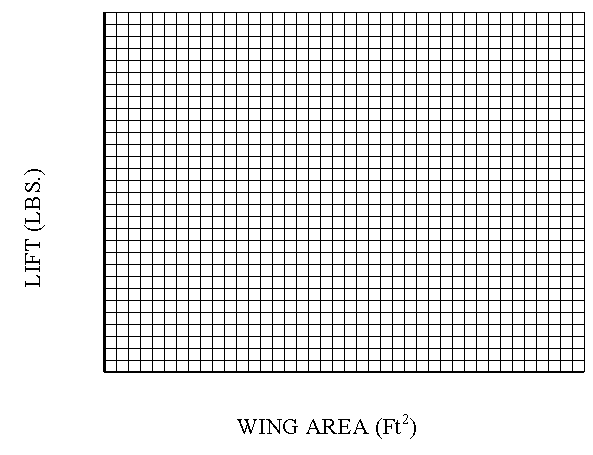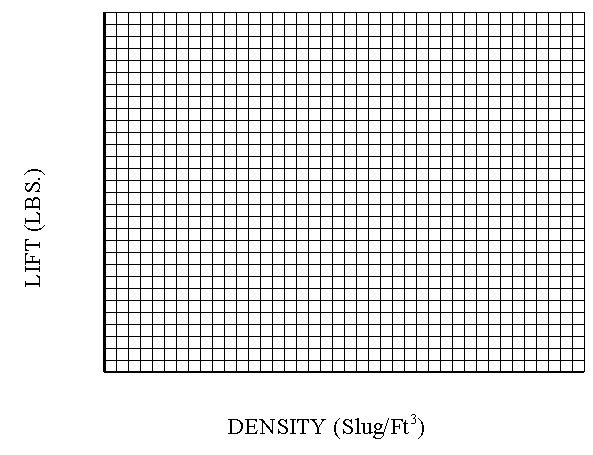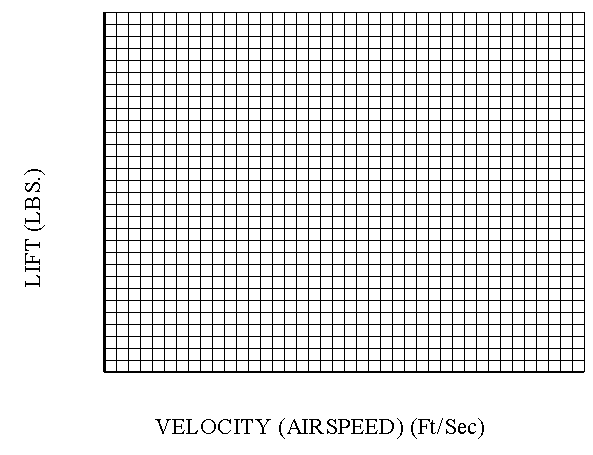PRINT THIS ANSWER SHEET TO FILL OUT AND TURN IN.

NAME_________________________________ CLASS____________________ DATE____________

## Lift Equation Worksheet

Step 1.

Using FoilSim, change the values for Area only. Pick 5 different values for the wing area. Record the values you used for area and the resulting lift.

 Wing Area (ft2) Lift (lbs)

Step 2.

Graph your recorded data for wing area and lift below.Step 3.

State the relationship between area and lift.

Step 4.

Using FoilSim, change the values for Altitude only. Reset the airfoil conditions to the original values given. Pick 5 different values for the altitude. Record the resulting values for density and the resulting lift.

 Density (slug/ft3) Lift (lbs)

Step 5.

Graph your recorded data for density and lift below.Step 6.

State the relationship between density and lift.

Step 7.

Using FoilSim, change the values for Velocity (airspeed) only. Reset the airfoil conditions to the original values given. Pick 5 different values for the velocity. Record the values you used for velocity and the resulting lift.

 Velocity (ft/sec) Lift (lbs)

Step 8.

Graph your recorded data for velocity (airspeed) and lift below.Step 9.

State the relationship between the velocity and the lift.

Step 10.

Using the lift equation, calculate the lift generated by an aircraft flying at 10,000 ft. at a speed of 120 ft./sec. The wing area is 500 ft.2 and the lift coefficient is 1.67. (You will need to use FoilSim or your graph to find the density at the given altitude.)

Step 11.

Calculate the lift coefficient for an aircraft with a 240 ft.2 wing area and a speed of 190ft./sec. that can generate 9530 lbs. of lift in air with a density of 0.00165 slug/ft3.

Step 12.

Find the velocity of an aircraft that generates 30,590 lbs. of lift when the wing area is 800 ft2. The lift coefficient for the aircraft is 1.4 and the air density is 0.00089 slug/ft3.

Step 13.

Using the graph you constructed in Step 2, find the lift produced by a wing area of 1200 ft2.

Step 14.

Using your graph from Step 5 and FoilSim, find the altitude for an aircraft that generates a lift of 15.39 lbs. (Remember: Set the airfoil conditions to the original settings.)